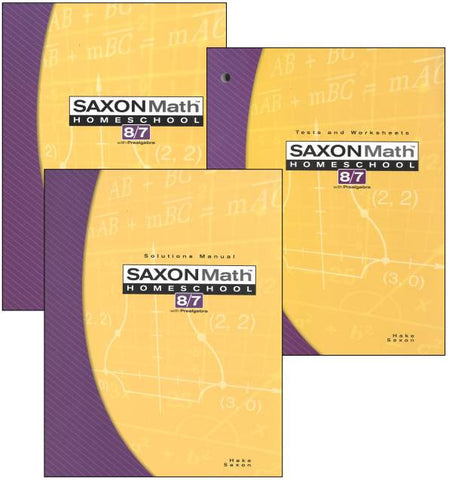# Saxon Math 8/7 3ED Homeschool KIT

• \$ 87.00
• Save \$ -8700

﻿FREE SHIPPING for purchase* Use code SAXONFREE

Saxon Math 8/7 Complete Homeschool Kit﻿

Building upon the principles taught in Saxon Math 7/6, the Saxon 8/7 textbook reviews arithmetic calculation, measurements, geometry and other skills, and introduces pre-algebra, ratios, probability and statistics. Students will specifically learn about adding/subtracting/multiplying fractions, equivalent fractions, the metric system, repeating decimals, scientific notation, Pi, graphing inequalities, multiplying algebraic terms, the Pythagorean Theorem, the slope-intercept form of linear equations, and more.

Lessons contain a warm-up (with facts practice, mental math, & problem-solving exercises); introduction to the new concept, lesson practice exercises where the new skill is practiced, and mixed practice exercises, which includes 25-30 old and new problems. In-depth "Investigations" are provided every 10 lessons and have their own set of questions.

*Free shipping is not offered for rental